# A hitchhiker guide to Matching Pursuit

The Matching Pursuit algorithm is popular in signal processing and applies well to digital images.

I have contributed a python implementation and we will show here how we may use that for extracting a sparse set of edges from an image.

```@inbook{Perrinet15bicv,
title = {Sparse models},
author = {Perrinet, Laurent U.},
booktitle = {Biologically-inspired Computer Vision},
chapter = {13},
editor = {Keil, Matthias and Crist\'{o}bal, Gabriel and Perrinet, Laurent U.},
publisher = {Wiley, New-York},
year = {2015}
}
```

#### setting things up¶

We will take some "baby steps first to show how it works, and then apply that to an image .

At first, we will define the SparseEdges framework which will create the necessary processing steps, from the raw image, to creating the multi-resolution scheme and the Matching Pursuit algorithm:

In :
```from SparseEdges import SparseEdges
mp = SparseEdges('https://raw.githubusercontent.com/bicv/SparseEdges/master/default_param.py')
mp.pe.N = 4
mp.pe.MP_alpha = 1.
mp.pe.do_whitening = False
```

This defines the following set of parameters:

In :
```print('Default parameters: ', mp.pe)
```
```Default parameters:  {'verbose': 0, 'N_image': None, 'seed': 42, 'N_X': 256, 'N_Y': 256, 'noise': 0.33, 'do_mask': False, 'mask_exponent': 3.0, 'do_whitening': False, 'white_name_database': 'kodakdb', 'white_n_learning': 0, 'white_N': 0.07, 'white_N_0': 0.0, 'white_f_0': 0.4, 'white_alpha': 1.4, 'white_steepness': 4.0, 'white_recompute': False, 'base_levels': 1.618, 'n_theta': 24, 'B_sf': 0.4, 'B_theta': 0.17453277777777776, 'N': 4, 'MP_alpha': 1.0, 'd_width': 45.0, 'd_min': 0.5, 'd_max': 2.0, 'N_r': 6, 'N_Dtheta': 24, 'N_phi': 12, 'N_scale': 5, 'loglevel_max': 7, 'edge_mask': True, 'do_rank': False, 'scale_invariant': True, 'multiscale': True, 'kappa_phase': 0.0, 'weight_by_distance': True, 'svm_n_jobs': 1, 'svm_test_size': 0.2, 'N_svm_grid': 32, 'N_svm_cv': 50, 'C_range_begin': -5, 'C_range_end': 10.0, 'gamma_range_begin': -14, 'gamma_range_end': 3, 'svm_KL_m': 0.34, 'svm_tol': 0.001, 'svm_max_iter': -1, 'svm_log': False, 'svm_norm': False, 'figpath': 'results', 'do_edgedir': False, 'edgefigpath': 'results/edges', 'matpath': 'data_cache', 'edgematpath': 'data_cache/edges', 'datapath': 'database', 'figsize': 14.0, 'figsize_hist': 8, 'figsize_cohist': 8, 'formats': ['png', 'pdf', 'jpg'], 'dpi': 450, 'use_cache': True, 'scale': 0.8, 'scale_circle': 0.08, 'scale_chevrons': 2.5, 'line_width': 1.0, 'line_width_chevrons': 0.75, 'edge_scale_chevrons': 180.0}
```

The useful imports for a nice notebook:

In :
```import matplotlib
matplotlib.rcParams.update({'text.usetex': False})
%matplotlib inline
%config InlineBackend.figure_format='retina'
import matplotlib.pyplot as plt

import numpy as np
np.set_printoptions(precision=4)#, suppress=True)

fig_width = 14
```
In :
```print('Range of spatial frequencies: ', mp.sf_0)
```
```Range of spatial frequencies:  [0.4545 0.2935 0.1895 0.1224 0.079  0.051  0.0329 0.0213 0.0137 0.0089
0.0057]
```
In :
```print('Range of angles (in degrees): ', mp.theta*180./np.pi)
```
```Range of angles (in degrees):  [-82.5 -75.  -67.5 -60.  -52.5 -45.  -37.5 -30.  -22.5 -15.   -7.5   0.
7.5  15.   22.5  30.   37.5  45.   52.5  60.   67.5  75.   82.5  90. ]
```

#### testing one step of (Matching + Pursuit)¶

Here, we will synthesize an image using 2 log-Gabor filters and check that they are correctly retrieved using a few Matching Pursuit steps. An edge will be represented by a `nd.array` with respectively `[x position, y center position, indice of angle in multi resolution, index of scale in multi resolution scheme]` and a coefficient (a complex number whose argument determines the phase of the log-Gabor filter):

In :
```# one
edge_in, C_in= [3*mp.pe.N_X/4, mp.pe.N_Y/2, 2, 2], 42
# the second
edge_bis, C_bis = [mp.pe.N_X/8, mp.pe.N_Y/4, 8, 4], 4.*np.sqrt(2)*np.exp(1j*np.pi/4.)
```

Which results in

In :
```# filters in Fourier space
FT_lg_in = mp.loggabor(edge_in, edge_in, sf_0=mp.sf_0[edge_in],
B_sf=mp.pe.B_sf, theta= mp.theta[edge_in], B_theta=mp.pe.B_theta)
FT_lg_bis = mp.loggabor(edge_bis, edge_bis, sf_0=mp.sf_0[edge_bis],
B_sf=mp.pe.B_sf, theta= mp.theta[edge_bis], B_theta=mp.pe.B_theta)
# mixing both and shows one
FT_lg_ = C_in *  FT_lg_in + C_bis * FT_lg_bis
image = mp.invert(FT_lg_)

_ = mp.show_FT(FT_lg_, axis=True)
```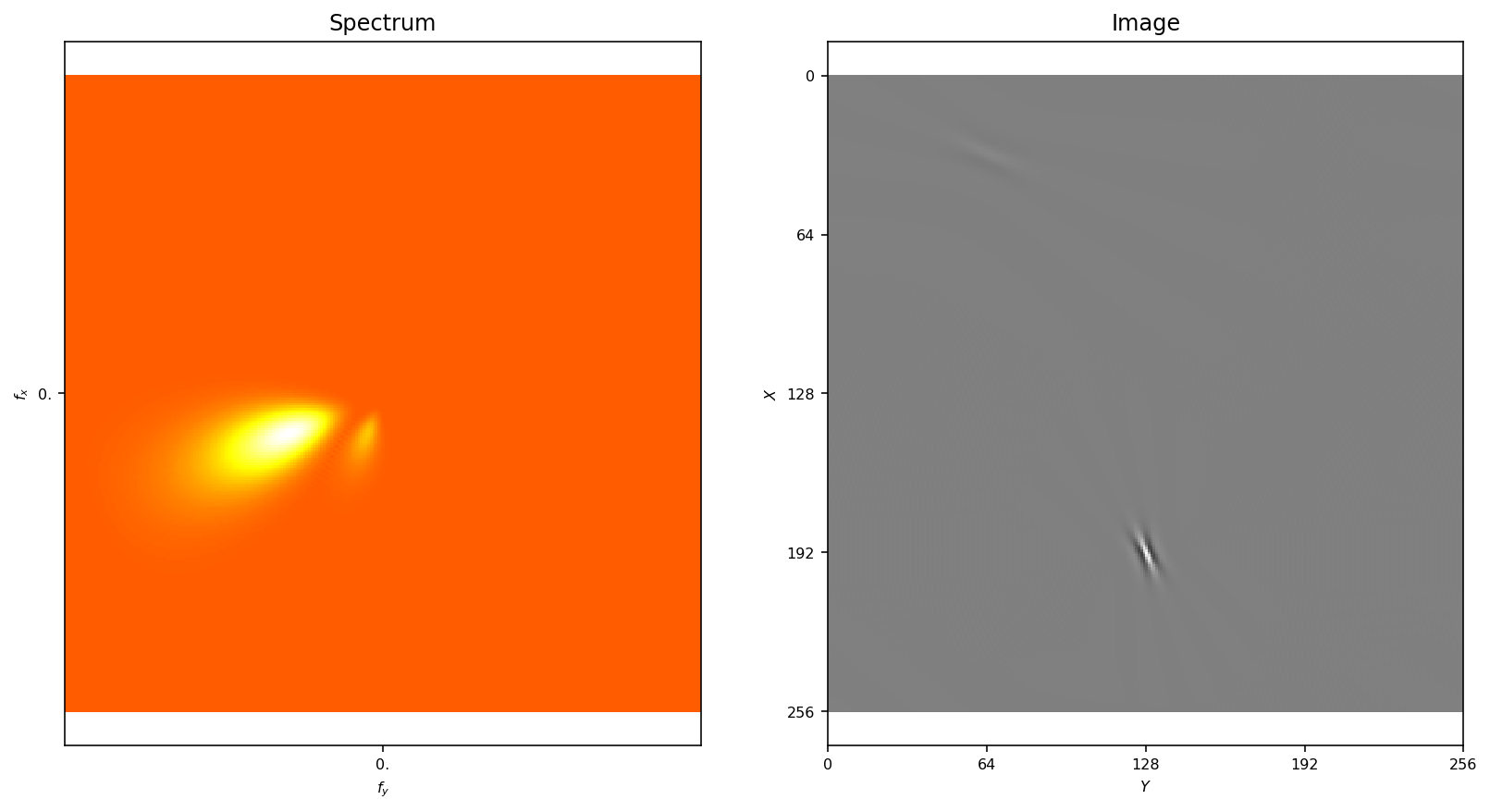We may start by computing the linear coefficients in the multi resolution scheme

In :
```C = mp.linear_pyramid(image)
```

These coefficients correspond to a measure of how well the image matches with the filters in the multi-resolution scheme. These will be used in the Matching step. We then detect the best match corresponding to the coefficient with maximum absolute activity:

In :
```edge_star = mp.argmax(C)
print('Coordinates of the maximum ', edge_star, ', true value: ', edge_in)
print('Value of the maximum ', C[edge_star], ', true value: ', C_in)
```
```Coordinates of the maximum  (192, 128, 2, 2) , true value:  [192.0, 128.0, 2, 2]
Value of the maximum  (41.99999999999932+4.213693403441275e-13j) , true value:  42
```

At this particular orientation and scale, the absolute activity looks like:

In :
```fig, a1, a2 = mp.show_spectrum(np.absolute(C[:, :, edge_star, edge_star]), axis=True)
_ = a2.plot([edge_star], [edge_star], 'r*')
```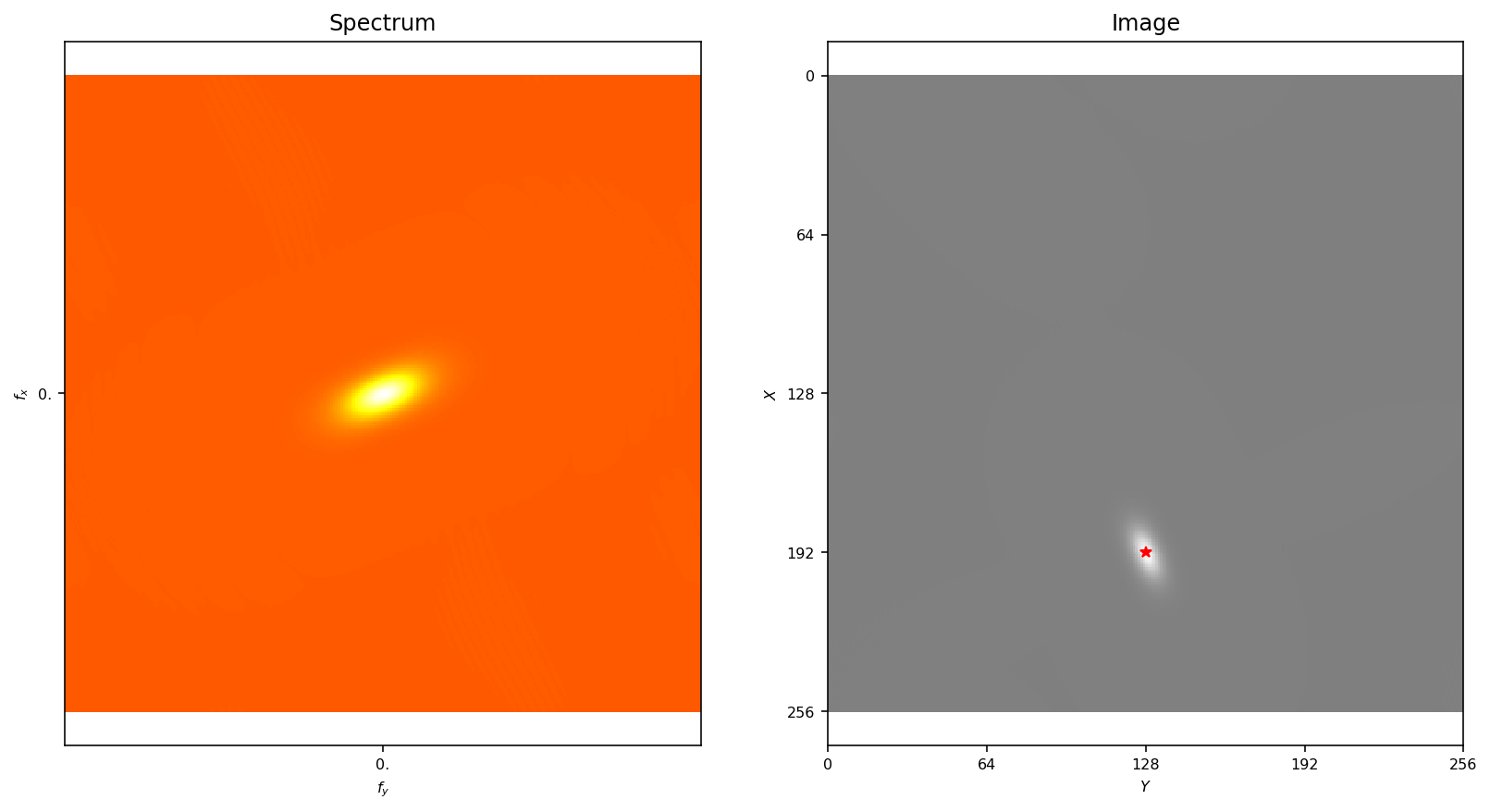On our image, this looks like:

In :
```fig, a1, a2 = mp.show_spectrum(image, axis=True)
_ = a2.plot([edge_star], [edge_star], 'r*')
```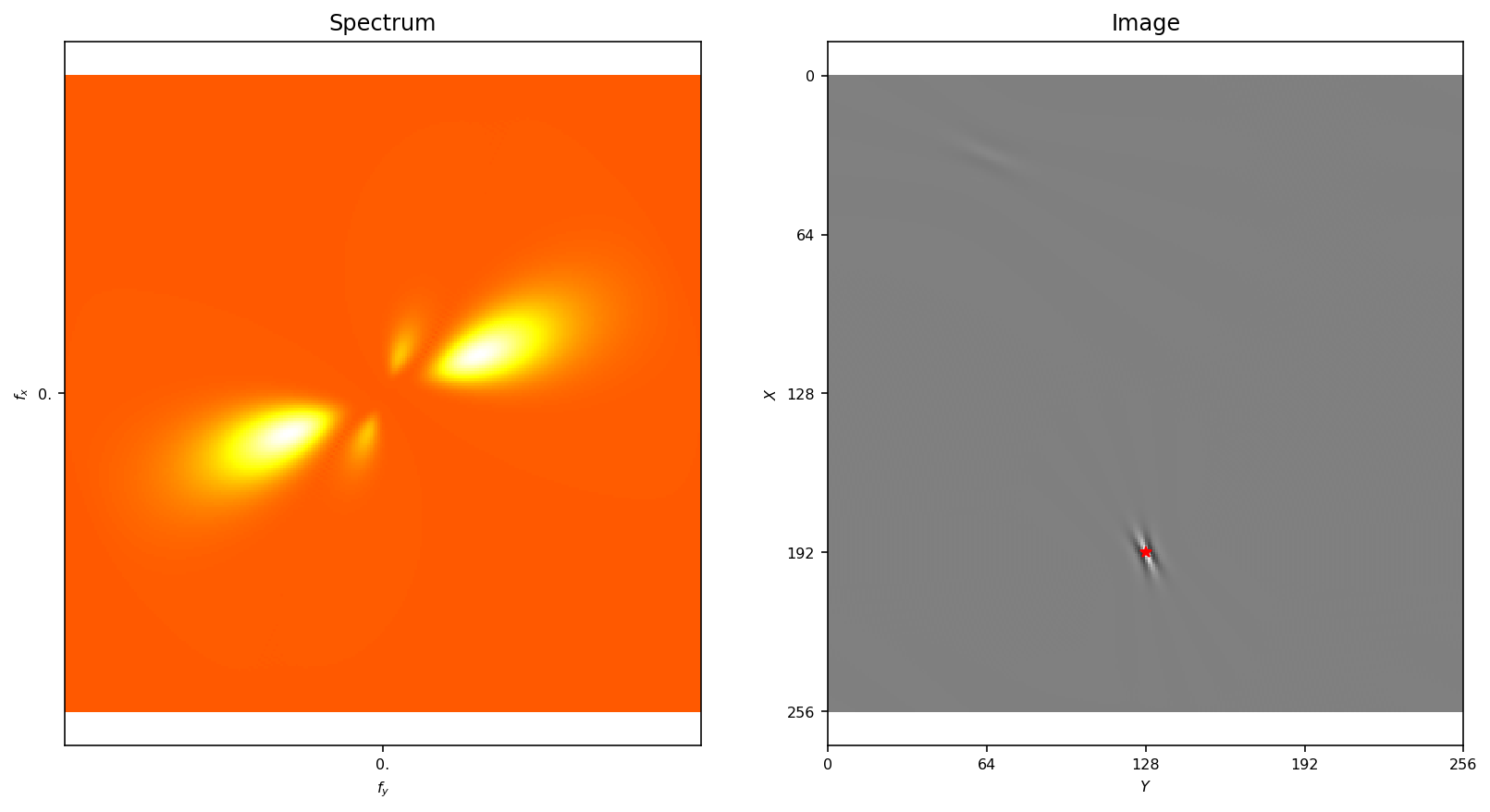Let's now extract and show the "winner" of the Matching Step:

In :
```FT_star = mp.loggabor(edge_star, edge_star, sf_0=mp.sf_0[edge_star],
B_sf=mp.pe.B_sf, theta= mp.theta[edge_star], B_theta=mp.pe.B_theta)
im_star = mp.invert(FT_star)
_ = mp.show_FT(FT_star, axis=True)
```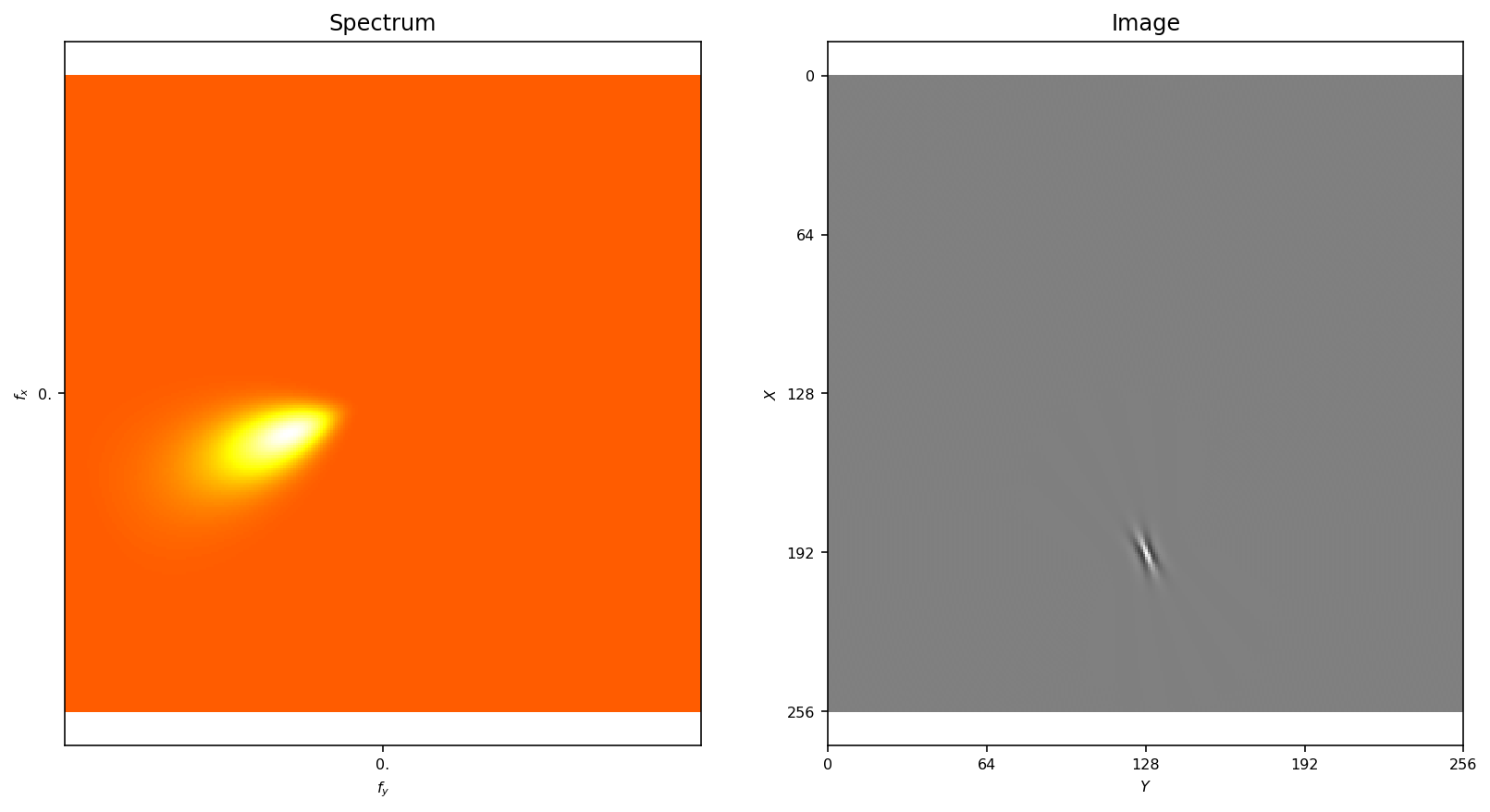Great, it looks like we have extracted the first edge. Let's first check that the energy of

• the sum the extracted component energy,
• maximum of its power spectrum (equivalent to the above, but we just check),
• half the mean spectrum energy (half? rememeber the trick used in Log-Gabor filters) are all equal to one:
In :
```print(np.sum(im_star**2), mp.FTfilter(im_star, FT_star).max(), np.mean(np.abs(FT_star)**2)/2)
```
```1.0000000000000002 1.0000000000000004 1.0000000000000004
```

Let's overlay the extracted edge on the image:

In :
```edge_star_in = np.array([edge_star, edge_star, mp.theta[edge_in], mp.sf_0[edge_star], np.absolute(C[edge_star]), np.angle(C[edge_star])])
mp.pe.figsize_edges = 9
fig, a = mp.show_edges(edge_star_in[:, np.newaxis], image=image)
```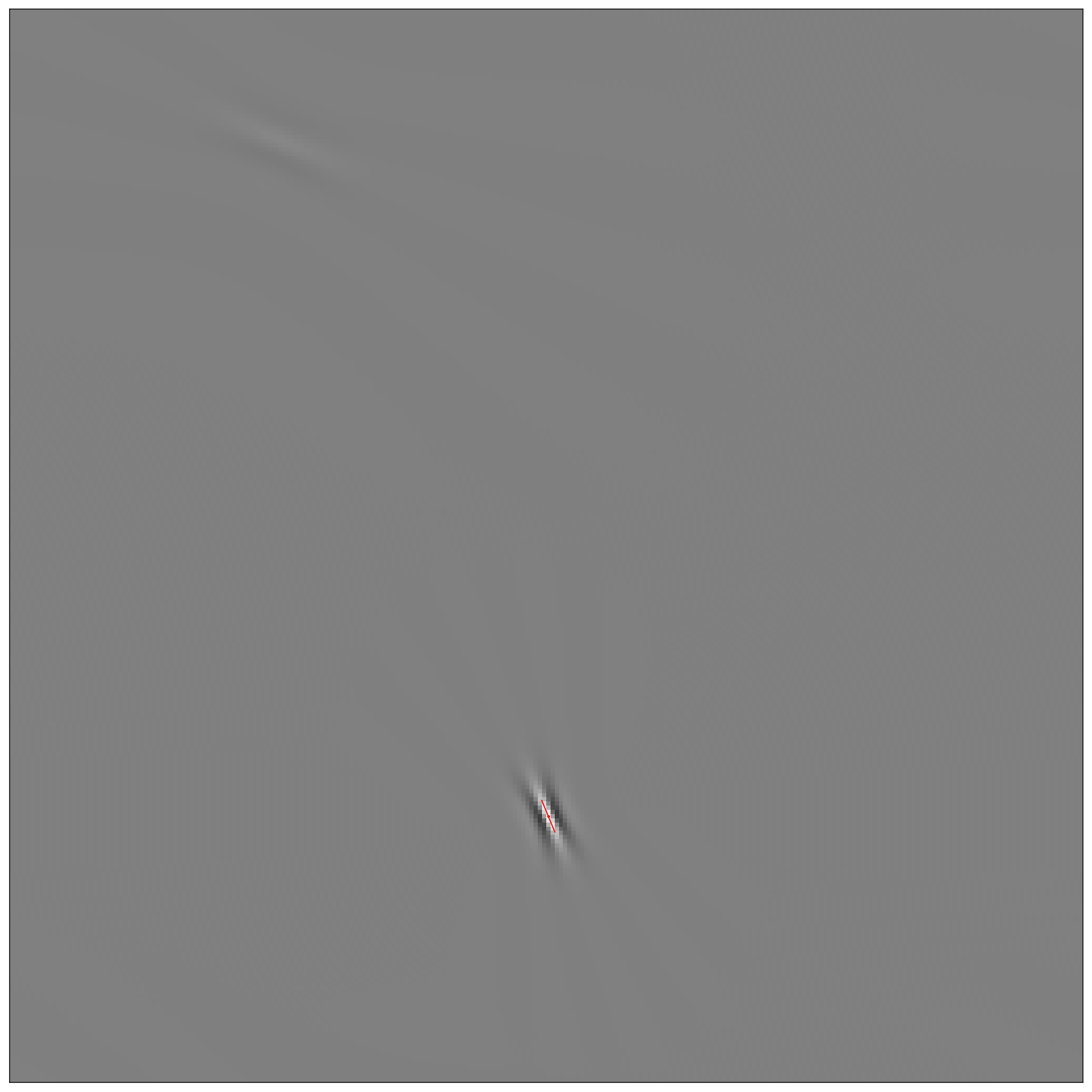Now, we may substract the residual from the image, it is the Pursuit step:

In :
```image_res = (image - C[edge_star] * im_star).real
fig, a1, a2 = mp.show_spectrum(image_res, axis=True)
_ = a2.plot([edge_star], [edge_star], 'r*')
```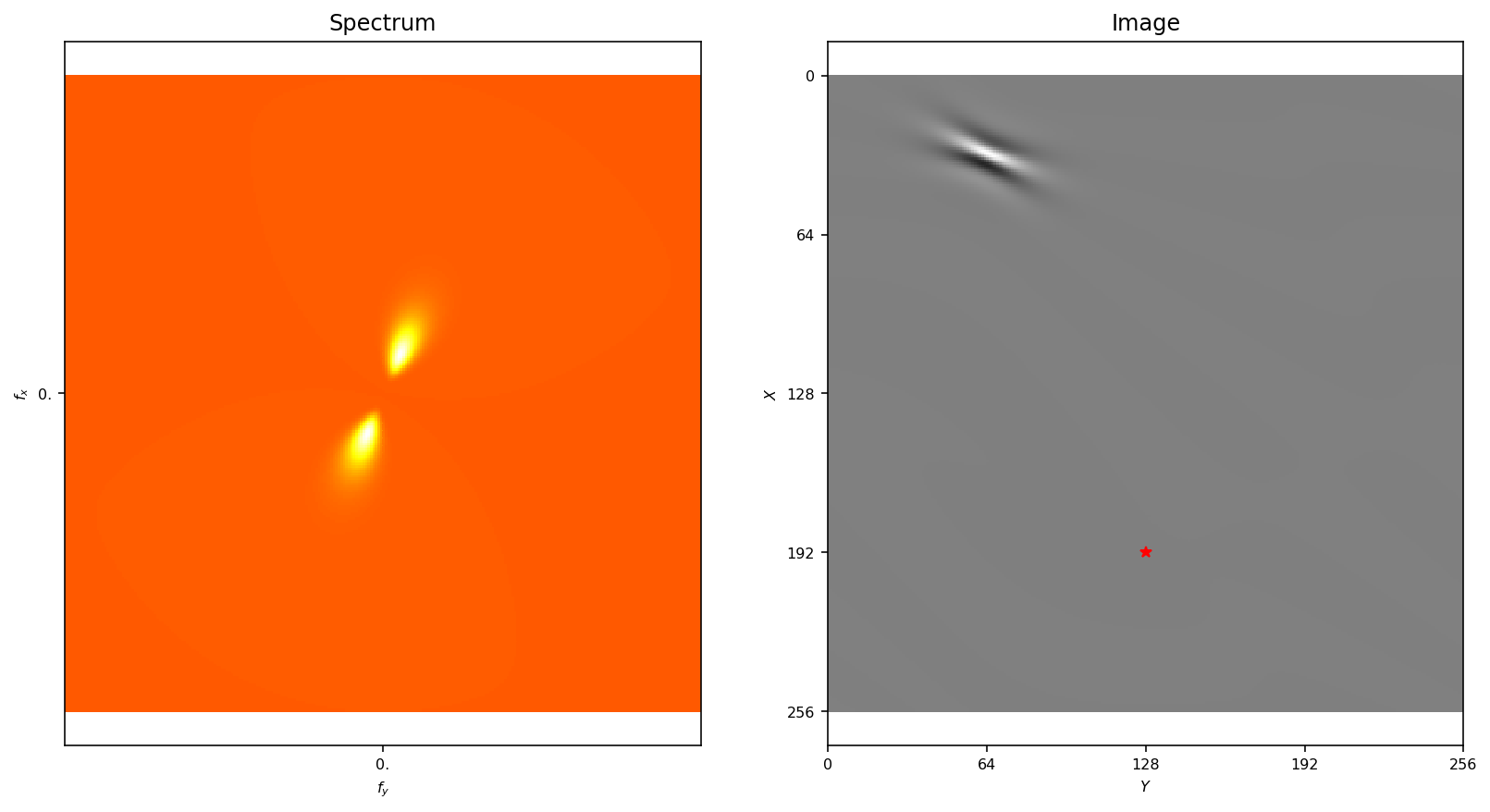Looks pretty clear now that only the second edge remains. We may now repeat another Matching step on the residual image:

In :
```C = mp.linear_pyramid(image_res)
edge_star_bis = mp.argmax(C)
print('Coordinates of the maximum ', edge_star_bis, ', true value: ', edge_bis)
print('Value of the maximum ', C[edge_star_bis], ', true value: ', C_bis)
```
```Coordinates of the maximum  (32, 64, 8, 4) , true value:  [32.0, 64.0, 8, 4]
Value of the maximum  (4+4.000000000000005j) , true value:  (4.000000000000001+4j)
```

Note: to store the complex value of the coefficient, we use the fact that it is easy to transform a complex number to 2 reals and back:

In :
```z = np.sqrt(2)*np.exp(1j*np.pi/4.)
z_, z_p = np.absolute(z), np.angle(z)
print(z, z_*np.exp(1j*z_p))
```
```(1.0000000000000002+1j) (1.0000000000000002+1j)
```

Note that the linear coefficients corresponding to the first winning filter are canceled:

In :
```print('Value of the residual ', C[edge_star], ', initial value: ', C_in)
```
```Value of the residual  (-1.1652065246228562e-14+5.182340906836718e-13j) , initial value:  42
```

Let's overlay the extracted edge on the image:

In :
```fig, a1, a2 = mp.show_spectrum(image_res, axis=True)
_ = a2.plot([edge_star_bis], [edge_star_bis], 'r*')
```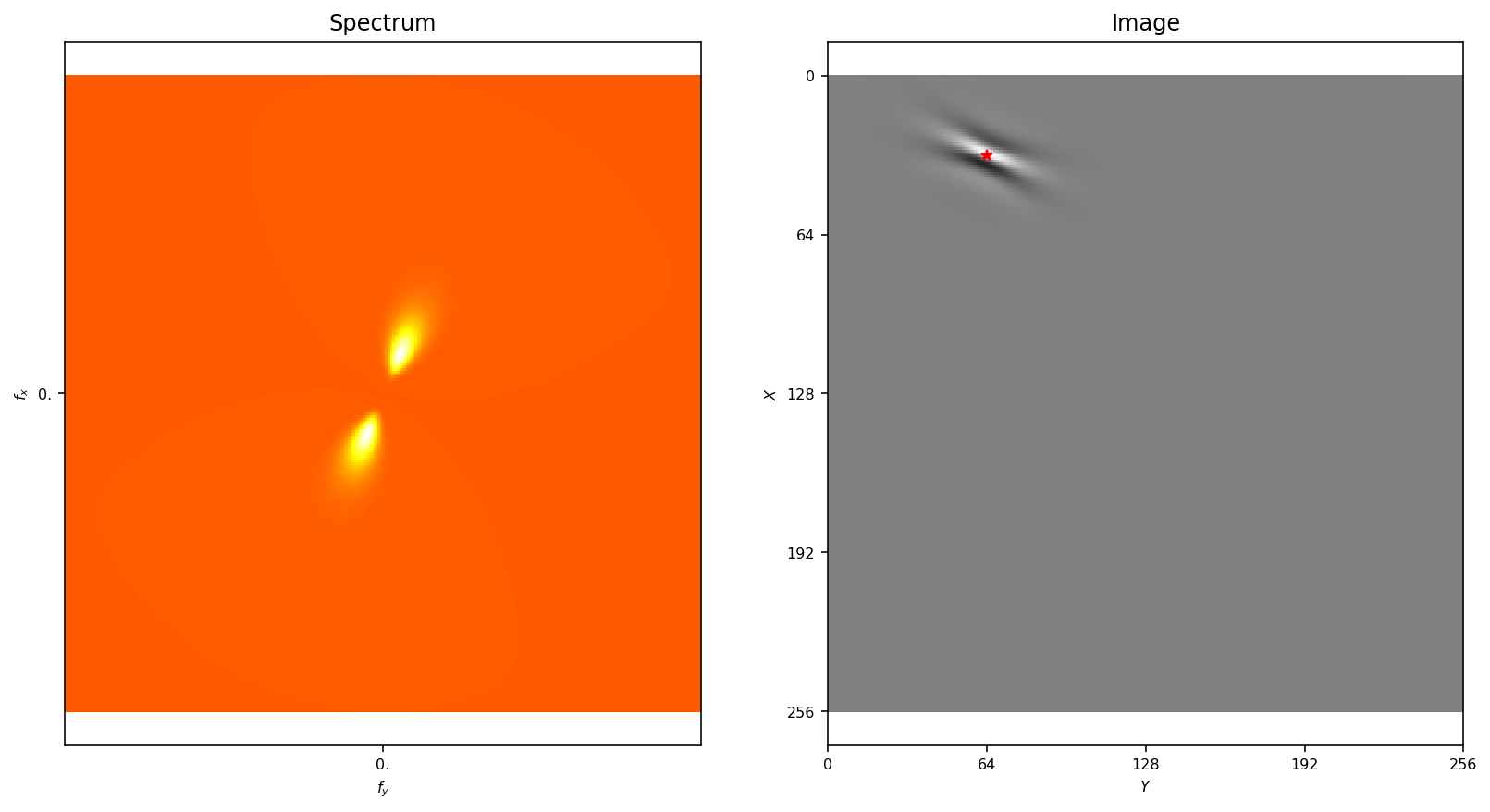We have well extracted the two edges:

In :
```edge_stars = np.vstack((edge_star_in,
np.array([edge_star_bis, edge_star_bis, mp.theta[edge_star_bis], mp.sf_0[edge_star_bis], np.absolute(C[edge_star_bis]), np.angle(C[edge_star_bis])])))
print(edge_stars)
mp.pe.figsize_edges = 9
fig, a = mp.show_edges(edge_stars.T, image=image)
```
```[[ 1.9200e+02  1.2800e+02 -1.1781e+00  1.8950e-01  4.2000e+01  1.0033e-14]
[ 3.2000e+01  6.4000e+01 -3.9270e-01  7.9005e-02  5.6569e+00  7.8540e-01]]
```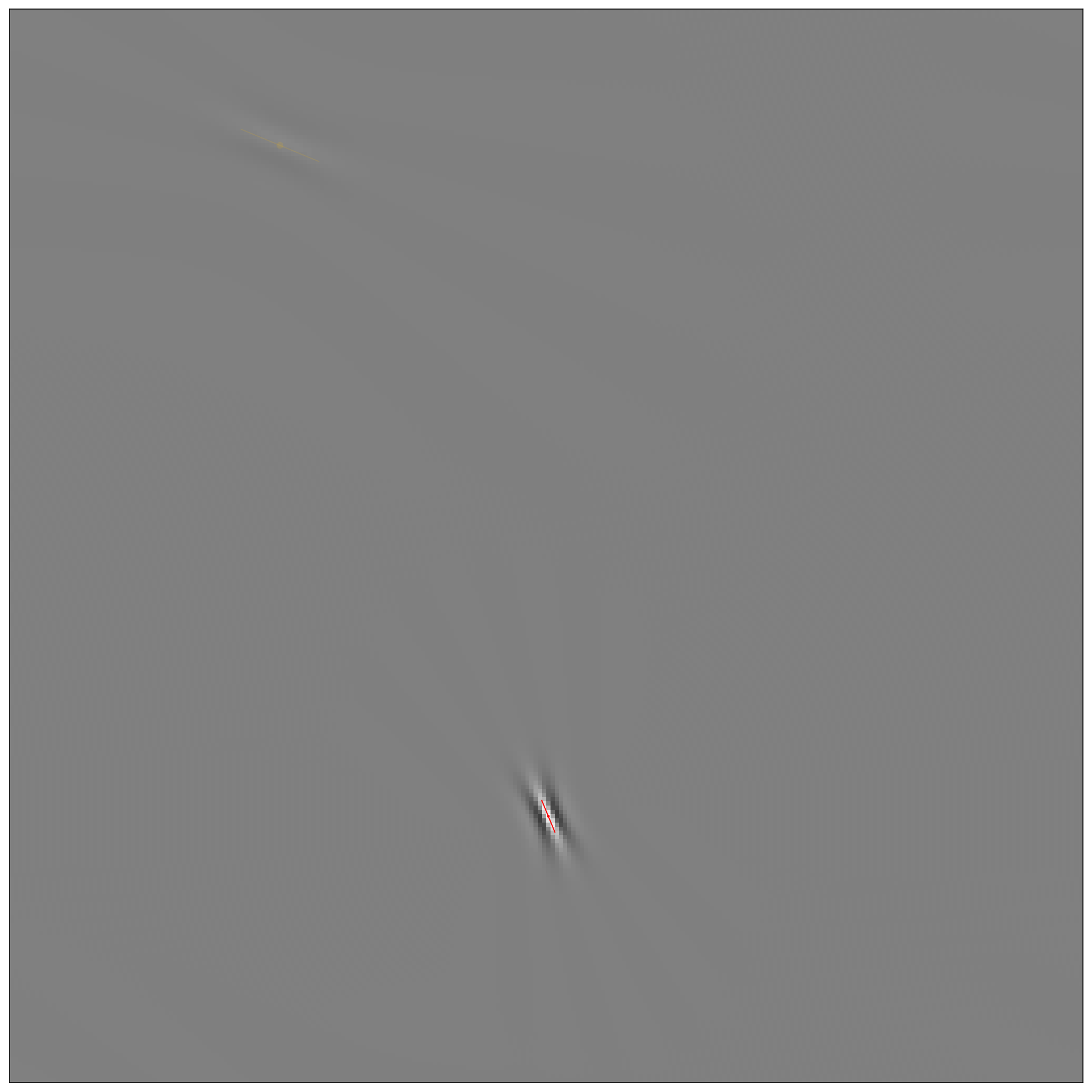#### testing four steps of Matching Pursuit¶

Let's redo these steps using the `run_mp` function:

In :
```# filters in Fourier space
FT_lg_in = mp.loggabor(edge_in, edge_in, sf_0=mp.sf_0[edge_in],
B_sf=mp.pe.B_sf, theta= mp.theta[edge_in], B_theta=mp.pe.B_theta)
FT_lg_bis = mp.loggabor(edge_bis, edge_bis, sf_0=mp.sf_0[edge_bis],
B_sf=mp.pe.B_sf, theta= mp.theta[edge_bis], B_theta=mp.pe.B_theta)
# mixing both and shows one
FT_lg_ = C_in *  FT_lg_in + C_bis * FT_lg_bis
```
In :
```fig = mp.show_FT(FT_lg_)
```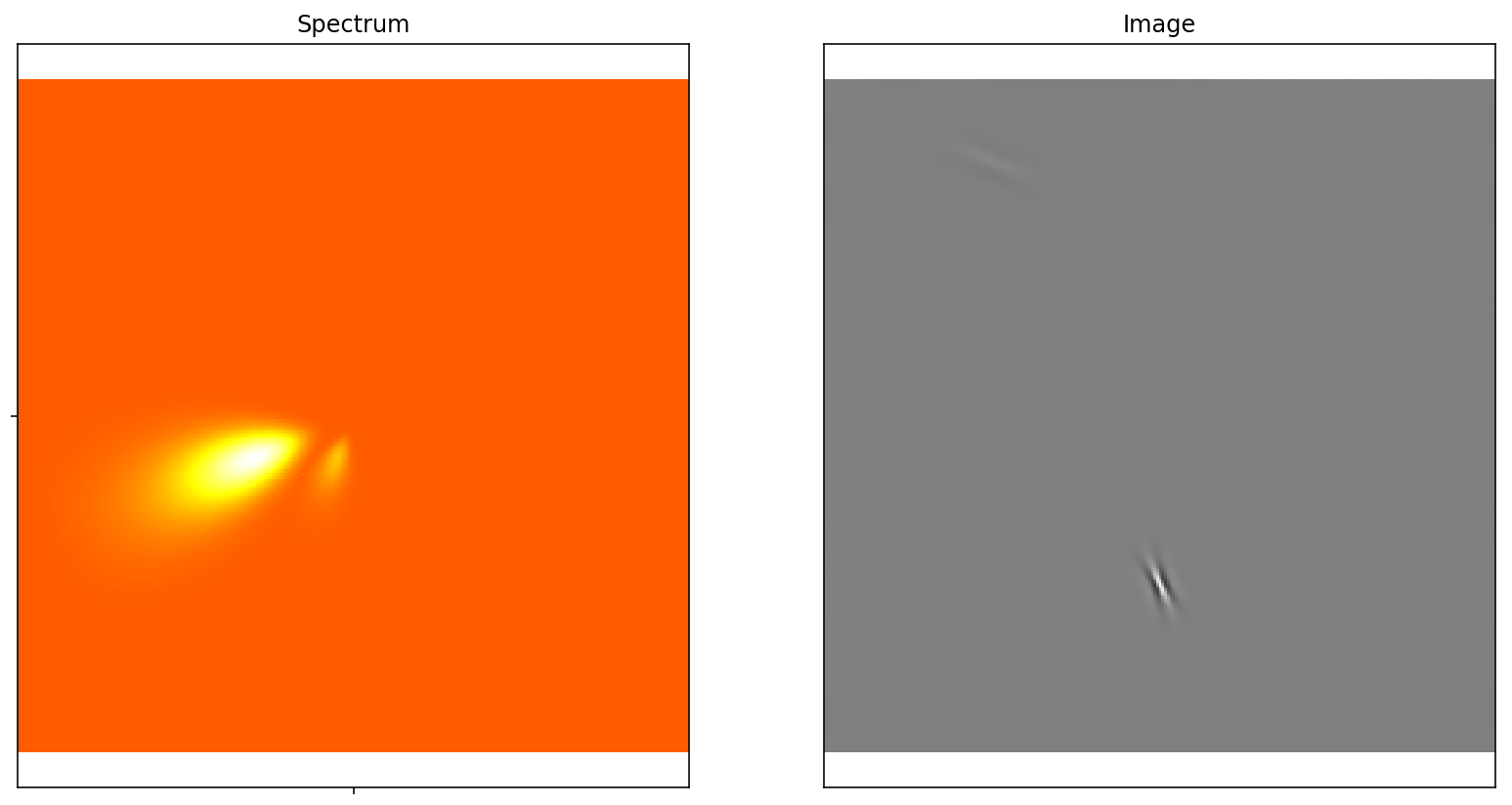In :
```image = mp.invert(FT_lg_)
edges, C_res = mp.run_mp(image, verbose=True)
```
```Edge  0 / 4  - Max activity  :  0.000  phase=  98.412  deg,  @  (192, 128, 2, 2)
Edge  1 / 4  - Max activity  :  0.000  phase=  -78.690  deg,  @  (32, 64, 8, 4)
Edge  2 / 4  - Max activity  :  0.000  phase=  67.620  deg,  @  (192, 128, 2, 2)
Edge  3 / 4  - Max activity  :  0.000  phase=  -96.698  deg,  @  (190, 127, 2, 2)
```
In :
```fig, a = mp.show_edges(edges, image=image)
```#### Testing edge detection on a natural image¶

trying out on a flikr image from @Doug88888:

In :
```!curl https://farm7.staticflickr.com/6058/6370387703_5e718ea681_q_d.jpg -o ../files/2015-05-22-a-hitchhiker-guide-to-matching-pursuit/6370387703_5e718ea681_q_d.jpg
```
```  % Total    % Received % Xferd  Average Speed   Time    Time     Time  Current
100 10602  100 10602    0     0  18814      0 --:--:-- --:--:-- --:--:-- 18831
```
In :
```%ls -l ../files/2015-05-22-a-hitchhiker-guide-to-matching-pursuit/MPtutorial*
```
```-rw-r--r--  1 laurentperrinet  staff   98432 Jun 25 14:17 ../files/2015-05-22-a-hitchhiker-guide-to-matching-pursuit/MPtutorial.npy
-rw-r--r--  1 laurentperrinet  staff   16512 Jun 25 14:17 ../files/2015-05-22-a-hitchhiker-guide-to-matching-pursuit/MPtutorial_MSE.npy
-rw-r--r--  1 laurentperrinet  staff   98432 Jun 18 18:26 ../files/2015-05-22-a-hitchhiker-guide-to-matching-pursuit/MPtutorial_lena.npy
-rw-r--r--  1 laurentperrinet  staff  145757 Jun 26 12:05 ../files/2015-05-22-a-hitchhiker-guide-to-matching-pursuit/MPtutorial_rec.gif
-rw-r--r--  1 laurentperrinet  staff   84303 Jun 26 12:05 ../files/2015-05-22-a-hitchhiker-guide-to-matching-pursuit/MPtutorial_rec.mp4
```
%rm ../files/2015-05-22-a-hitchhiker-guide-to-matching-pursuit/MPtutorial.npy ../files/2015-05-22-a-hitchhiker-guide-to-matching-pursuit/MPtutorial_MSE.npy%rm ../files/2015-05-22-a-hitchhiker-guide-to-matching-pursuit/MPtutorial_MSE.npy
In :
```import numpy as np
from SparseEdges import SparseEdges
mp = SparseEdges('https://raw.githubusercontent.com/bicv/SparseEdges/master/default_param.py')
if not mp.pe.do_whitening: print('\!/ Wrong parameters... \!/')

# where should we store the data + figures generated by this notebook
import os
name = 'MPtutorial'
mp.pe.matpath, mp.pe.figpath = '../files/2015-05-22-a-hitchhiker-guide-to-matching-pursuit', '../files/2015-05-22-a-hitchhiker-guide-to-matching-pursuit'

mp.pe.N = 2048
mp.pe.MP_alpha = 1.
mp.pe.MP_alpha = .8

mp.init()

# defining input image (get it @ https://www.flickr.com/photos/doug88888/6370387703)

white = mp.pipeline(image, do_whitening=True)

import os
matname = os.path.join(mp.pe.matpath, name + '.npy')

try:
except:
edges, C_res = mp.run_mp(white, verbose=True)
np.save(matname, edges)

matname_MSE = os.path.join(mp.pe.matpath, name + '_MSE.npy')
try:
except:
MSE = np.ones(mp.pe.N)
image_rec = np.zeros_like(image)
for i_N in range(mp.pe.N):
MSE[i_N] =  ((white-image_rec)**2).sum()
image_rec += mp.reconstruct(edges[:, i_N][:, np.newaxis])

np.save(matname_MSE, MSE)
```

Showing the original image with the edges overlaid:

In :
```#edges = np.load(matname)

fig_width_pt = 318.670  # Get this from LaTeX using \showthe\columnwidth
inches_per_pt = 1.0/72.27               # Convert pt to inches
fig_width = fig_width_pt*inches_per_pt  # width in inches
mp.pe.figsize_edges = .382 * fig_width  # useful for papers
mp.pe.figsize_edges = 9 # useful in notebooks
mp.pe.line_width = 1.
mp.pe.scale = 1.
fig, a = mp.show_edges(edges, image=image, show_phase=True, show_mask=True)
#mp.savefig(fig, name + '_rec')
```